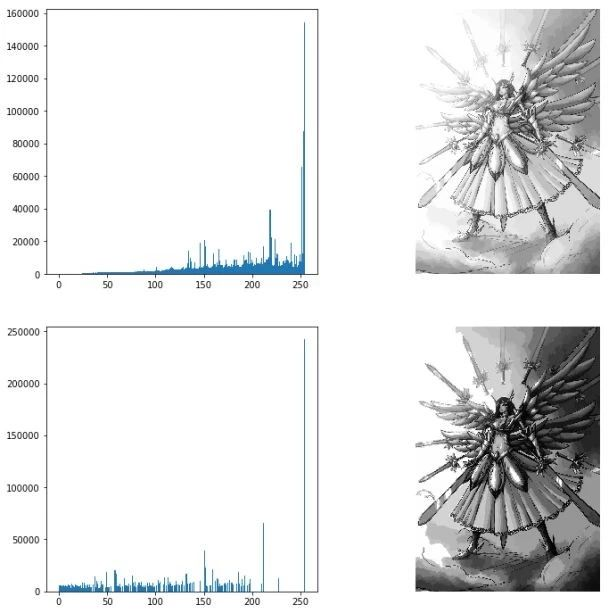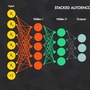## 实战 | 用Python做图像处理（三）

2018 年 5 月 29 日 七月在线实验室Numpy的数组对象是我们处理图像和数据的主要工具。想要对图像进行缩放处理没有现成的简单的方法。因此我们使用如下代码实现图像的缩放：

`from PIL import Imagefrom numpy import *from pylab import *def imresize(im, sz):    pil_im = Image.fromarray(uint8(im))    return array(pil_im.resize(sz))im = Image.open("test.jpg")im1 = imresize(im, (128, 128))figure()gray()subplot(1, 2, 1)imshow(im)subplot(1, 2, 2)imshow(im1)title("Zoom picture")show()`

图像均匀操作是减少图像噪声的一种简单方式，通常用于艺术特效，我们可以简单的从图像列表中计算出一幅平均图像，假设所有的图像具有相同的大小，我们可以将这些图像简单的相加，然后除以图像的数目，来计算平均图像，下面的函数可以用来计算平均图像：

`def compute_average(imlist):    averageim = array(Image.open(imlist), 'f')    for imname in imlist[1:]:        try:            averageim += array(Image.open(imname))        except:            print(imname + '...skipped')    averageim /= len(imlist)return array(averageim, 'unit8')`

`from PIL import Imageimport numpy as npfrom pylab import *import matplotlib.pyplot as pltimport osdef compute_average(imlist):    """计算图像列表的平均像素"""    # 打开第一幅图像，将其存储在浮点型数组中    averageim = array(Image.open(imlist), 'f')    for imname in imlist[1:]:        try:            averageim += array(Image.open(imname))        except:            print            imname + "...skipped"        averageim /= len(imlist)    return array(averageim, 'uint8')if __name__ == "__main__":    dirs = os.listdir("/home/xuna/桌面/image")    imlist = []    for name in dirs:        name = "/home/xuna/桌面/image/" + name        imlist.append(name)    print    imlist    im = compute_average(imlist)    imshow(im)    show()`

r的概率密度为，s的概率密度为：

`import numpy as npfrom PIL import Imageimport matplotlib.pyplot as pltimport matplotlib.cm as cmdef histeq(image_array, image_bins=256):    # 将图像矩阵转化成直方图数据，返回元组(频数，直方图区间坐标)    image_array2, bins = np.histogram(image_array.flatten(), image_bins)    # 计算直方图的累积函数    cdf = image_array2.cumsum()    # 将累积函数转化到区间[0,255]    cdf = (255.0 / cdf[-1]) * cdf    # 原图像矩阵利用累积函数进行转化，插值过程    image2_array = np.interp(image_array.flatten(), bins[:-1], cdf)    # 返回均衡化后的图像矩阵和累积函数    return image2_array.reshape(image_array.shape), cdfimage = Image.open("test.jpg").convert("L")image_array = np.array(image)fig = plt.figure(figsize=(15, 15))ax = fig.add_subplot(2, 2, 1)ax.hist(image_array.flatten(), 256)plt.subplot(2, 2, 2)plt.imshow(image_array, cmap=cm.gray)plt.axis("off")a = histeq(image_array)  # 利用刚定义的直方图均衡化函数对图像进行均衡化处理plt.subplot(2, 2, 3)plt.hist(a.flatten(), 256)plt.subplot(2, 2, 4)plt.imshow(Image.fromarray(a), cmap=cm.gray)plt.axis("off")plt.show()`

ROF模型的数学基础和处理技巧非常高深，有兴趣的读者可以自学深入了解，本文只是做一个简单介绍。

ROF模型

`from numpy import *from numpy import linalg as LAfrom numpy import randomfrom scipy.ndimage import filtersfrom scipy.misc import imsavefrom PIL import Imagedef denoise(im, U_init, tolerance=0.1, tau=0.125, tv_weight=100):    m, n = im.shape  # 噪声图像的大小    U = U_init    Px = im  # 对偶域的x 分量    Py = im  # 对偶域的y 分量    error = 1    while (error > tolerance):        Uold = U        # 原始变量的梯度        GradUx = roll(U, -1, axis=1) - U  # 变量U 梯度的x 分量        GradUy = roll(U, -1, axis=0) - U  # 变量U 梯度的y 分量        # 更新对偶变量          PxNew = Px + (tau / tv_weight) * GradUx        PyNew = Py + (tau / tv_weight) * GradUy        NormNew = maximum(1, sqrt(PxNew ** 2 + PyNew ** 2))        Px = PxNew / NormNew  # 更新x 分量（对偶）        Py = PyNew / NormNew  # 更新y 分量（对偶）        # 更新原始变量        RxPx = roll(Px, 1, axis=1)        RyPy = roll(Py, 1, axis=0)        DivP = (Px - RxPx) + (Py - RyPy)  # 对偶域的散度        U = im + tv_weight * DivP  # 更新原始变量        # 更新误差        error = linalg.norm(U - Uold) / sqrt(n * m)    return U, im - Uim = zeros((500, 500))im[100:400, 100:400] = 128im[200:300, 200:300] = 255im = im + 30 * random.standard_normal((500, 500))U, T = denoise(im, im)G = filters.gaussian_filter(im, 10)from scipy.misc import imsaveimsave('test1.pdf', U)imsave('test2.pdf', G)`

（a）

（b）

`from numpy import *from numpy import linalg as LAfrom numpy import randomfrom scipy.ndimage import filtersfrom scipy.misc import imsavefrom PIL import Imageimport matplotlib.pyplot as pltdef denoise(im, U_init, tolerance=0.1, tau=0.125, tv_weight=100):    m, n = im.shape  # 噪声图像的大小    U = U_init    Px = im  # 对偶域的x 分量    Py = im  # 对偶域的y 分量    error = 1    while (error > tolerance):        Uold = U        GradUx = roll(U, -1, axis=1) - U  # 变量U 梯度的x 分量        GradUy = roll(U, -1, axis=0) - U  # 变量U 梯度的y 分量        PxNew = Px + (tau / tv_weight) * GradUx        PyNew = Py + (tau / tv_weight) * GradUy        NormNew = maximum(1, sqrt(PxNew ** 2 + PyNew ** 2))        Px = PxNew / NormNew  # 更新x 分量（对偶）        Py = PyNew / NormNew  # 更新y 分量（对偶）        RxPx = roll(Px, 1, axis=1)  # 对x 分量进行向右x 轴平移        RyPy = roll(Py, 1, axis=0)  # 对y 分量进行向右y 轴平移        DivP = (Px - RxPx) + (Py - RyPy)  # 对偶域的散度        U = im + tv_weight * DivP  # 更新原始变量        # 更新误差        error = linalg.norm(U - Uold) / sqrt(n * m)    return U, im - Ufig = plt.figure(figsize=(15, 15))im = array(Image.open('test.jpg').convert('L'))U, T = denoise(im, im)plt.imshow(U, plt.cm.gray)plt.axis('equal')plt.axis("off")plt.show()`

# 《机器学习 第九期》从零到机器学习实战项目，提供GPU&CPU双云平台，作业考试1V1批改（优秀学员内推BAT等）；点击文末“阅读原文”了解详情111

### 相关内容

IM：IFIP/IEEE International Symposium on Integrated Network Management。 Explanation：综合网络管理国际研讨会。 Publisher：IFIP/IEEE SIT： http://dblp.uni-trier.de/db/conf/im/index.html013001700300130080010003001100100060

64+阅读 · 2020年7月1日

134+阅读 · 2020年5月21日

116+阅读 · 2020年3月22日

96+阅读 · 2020年3月21日

230+阅读 · 2020年3月17日

117+阅读 · 2020年3月12日

Xiaopeng Zhang
12+阅读 · 2020年6月10日
Jing Li,Aixin Sun,Jianglei Han,Chenliang Li
24+阅读 · 2020年3月13日
Marco Menardi,Alex Falcon,Saida S. Mohamed,Lorenzo Seidenari,Giuseppe Serra,Alberto Del Bimbo,Carlo Tasso
3+阅读 · 2019年10月9日
Xintao Wang,Ke Yu,Shixiang Wu,Jinjin Gu,Yihao Liu,Chao Dong,Chen Change Loy,Yu Qiao,Xiaoou Tang
5+阅读 · 2018年9月17日
Anoop Cherian,Alan Sullivan
4+阅读 · 2018年7月12日
Hiroki Shimanaka,Tomoyuki Kajiwara,Mamoru Komachi
3+阅读 · 2018年5月18日
Jianxin Lin,Yingce Xia,Tao Qin,Zhibo Chen,Tie-Yan Liu
6+阅读 · 2018年5月1日
Jaakko Lehtinen,Jacob Munkberg,Jon Hasselgren,Samuli Laine,Tero Karras,Miika Aittala,Timo Aila
5+阅读 · 2018年3月12日
Yun Chen,Yang Liu,Victor O. K. Li
4+阅读 · 2018年2月9日
Zhou Yin,Wei-Shi Zheng,Ancong Wu,Hong-Xing Yu,Hai Wang,Jianhuang Lai
7+阅读 · 2018年2月6日
Top